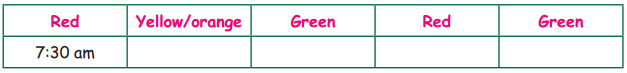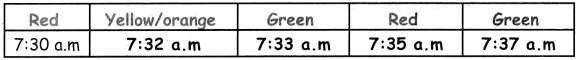Students can download 4th Maths Term 1 Chapter 3 Patterns Ex 3.4 Questions and Answers, Notes, Samacheer Kalvi 4th Maths Guide Pdf helps you to revise the complete Tamilnadu State Board New Syllabus, helps students complete homework assignments and to score high marks in board exams.

## Tamilnadu Samacheer Kalvi 4th Maths Solutions Term 1 Chapter 3 Patterns Ex 3.4

Fill in the blanks :

i. 90. 180, 270, _____, _____, _____,
ii. A9, B18, C27, 836, _____, _____, _____,
i. 90. 180, 270, _____, _____, _____,
360, 450, 540.

ii. A9, B18, C27, 836, _____, _____, _____,
E45 F54, G63.B. Circle the multiples of 9:
25, 27, 35, 36, 45, 46, 54, 55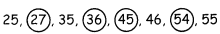C. Complete the following sequence:

Question 1.
125, 150, 175, _____, _____, _____,
200, 225, 250

Question 2.
100, 400, 700. _____, _____, _____,
1000, 1300, 1600Question 3.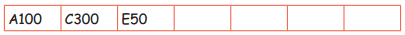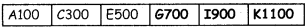Question 4.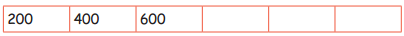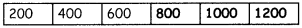Complete the following sequence.
Question 1.
9 × 6 = 54
9 × 66 = 594
9 × 666 = 5994
9 × 6666 = 5 ____ 4
9 × 666666 = ______
9 × 6666 = 59994
9 × 666666 =5999994

Question 2.
9 × 111 = 999
9 × 222 = 1998
9 × 333 = 2997
9 × 444 = ______
9 × 555 = ______
9 × 666 = ______
9 × 444 = 3996
9 × 555 = 4995
9 × 666 = 5994.Question 1.
The school bell rings once in an hour, to indicate that the session ends/next session begins. And for break, it will be 20 minutes. Shall we try to fill this up.
Here is the time table.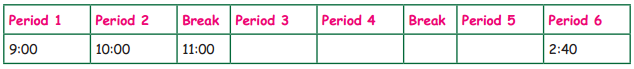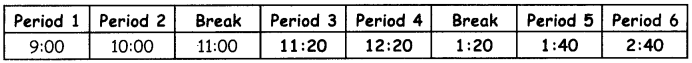Question 2.
Imagine you are a traffic inspector. You are asked to design the traffic signal timings. Can you design it?
Here is the time table.